# User:Tohline/H Book

(Difference between revisions)
 Revision as of 17:27, 17 January 2015 (view source)Tohline (Talk | contribs)← Older edit Revision as of 21:11, 26 February 2015 (view source)Tohline (Talk | contribs) (→Structure:: Begin adding summary text for spherical "structure")Newer edit → Line 37: Line 37: ===Structure:=== ===Structure:=== + + Here we show how the set of principal governing equations (PGEs) may be solved to determine the equilibrium structure of spherically symmetric fluid configurations — such as individual, nonrotating stars or interstellar gas clouds having a rather idealized geometry.  After supplementing the PGEs by specifying an equation of state of the fluid, the system of equations is usually solved by employing one of three techniques to obtain a "detailed force-balanced" model that provides the radius, $~R_\mathrm{eq}$, of the equilibrium configuration — given its mass, $~M$, and central pressure, $~P_c$, for example — as well as details regarding the internal radial profiles of the mass-density and fluid pressure.  As our various discussions illustrate, even a simple ''polytropic'' equation of state, that is, +
+ + +
+ $~P$ + + $~=$ + + $~K \rho^{(n+1)/n} \, ,$ +
+ + + + + + + gives rise to equilibrium configurations that have a wide variety of internal structural profiles. + + If one is not particularly concerned about details regarding the distribution of matter ''within'' the equilibrium configuration, a reasonably good estimate of the size of the equilibrium system can be determined by assuming a uniform-density structure then identifying extrema in the system's global free energy, that is, by identifying properties that satisfy the ''scalar virial theorem''.  Specifically, for ''isolated'' systems in virial equilibrium, the following relation between configuration parameters holds: +
+ + +
+ $~\frac{GM^2}{P_c R_\mathrm{eq}^4}$ + + $~=$ + + $~\biggl(\frac{2^2\cdot 5 \pi}{3} \biggr) \frac{\mathfrak{f}_A \cdot \mathfrak{f}_M^2}{\mathfrak{f}_W} + \, ,$ +
+ + + + + + + where, all three of the dimensionless ''structural form factors'', $~\mathfrak{f}_M$, $~\mathfrak{f}_W$, and $~\mathfrak{f}_A$, equal unity under the assumption that the equilibrium configuration has uniform density and uniform pressure throughout, while all three are ''of order'' unity otherwise.  If the configuration — such as an interstellar gas cloud — is not isolated but is, instead, embedded in a hot, tenuous external medium that exerts an external pressure, $~P_e$, the configuration's equilibrium parameters will be related via the expression, +
+ + +
+ $~\frac{GM^2}{P_c R_\mathrm{eq}^4}$ + + $~=$ + + $~\biggl(\frac{2^2\cdot 5 \pi}{3} \biggr) \frac{\mathfrak{f}_M^2}{\mathfrak{f}_W} \biggl[ \mathfrak{f}_A - \frac{P_e}{P_c} \biggr] + \, .$ +
+ + + + + + + + We have introduced these structural form factors into our discussion of the free energy of spherically symmetric, self-gravitating configurations because +

## Revision as of 21:11, 26 February 2015

Preface from the original version of this HyperText Book (H_Book):

November 18, 1994

Much of our present, basic understanding of the structure, stability, and dynamical evolution of individual stars, short-period binary star systems, and the gaseous disks that are associated with numerous types of stellar systems (including galaxies) is derived from an examination of the behavior of a specific set of coupled, partial differential equations. These equations — most of which also are heavily utilized in studies of continuum flows in terrestrial environments — are thought to govern the underlying physics of all macroscopic "fluid" systems in astronomy. Although relatively simple in form, they prove to be very rich in nature... <more>

# Applications

## Spherically Symmetric Configurations

### Structure:

Here we show how the set of principal governing equations (PGEs) may be solved to determine the equilibrium structure of spherically symmetric fluid configurations — such as individual, nonrotating stars or interstellar gas clouds having a rather idealized geometry. After supplementing the PGEs by specifying an equation of state of the fluid, the system of equations is usually solved by employing one of three techniques to obtain a "detailed force-balanced" model that provides the radius,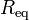$~R_\mathrm{eq}$, of the equilibrium configuration — given its mass,$~M$, and central pressure,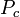$~P_c$, for example — as well as details regarding the internal radial profiles of the mass-density and fluid pressure. As our various discussions illustrate, even a simple polytropic equation of state, that is,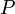$~P$$~=$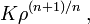$~K \rho^{(n+1)/n} \, ,$

gives rise to equilibrium configurations that have a wide variety of internal structural profiles.

If one is not particularly concerned about details regarding the distribution of matter within the equilibrium configuration, a reasonably good estimate of the size of the equilibrium system can be determined by assuming a uniform-density structure then identifying extrema in the system's global free energy, that is, by identifying properties that satisfy the scalar virial theorem. Specifically, for isolated systems in virial equilibrium, the following relation between configuration parameters holds: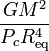$~\frac{GM^2}{P_c R_\mathrm{eq}^4}$$~=$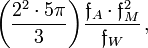$~\biggl(\frac{2^2\cdot 5 \pi}{3} \biggr) \frac{\mathfrak{f}_A \cdot \mathfrak{f}_M^2}{\mathfrak{f}_W} \, ,$

where, all three of the dimensionless structural form factors,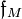$~\mathfrak{f}_M$,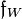$~\mathfrak{f}_W$, and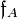$~\mathfrak{f}_A$, equal unity under the assumption that the equilibrium configuration has uniform density and uniform pressure throughout, while all three are of order unity otherwise. If the configuration — such as an interstellar gas cloud — is not isolated but is, instead, embedded in a hot, tenuous external medium that exerts an external pressure,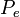$~P_e$, the configuration's equilibrium parameters will be related via the expression,$~\frac{GM^2}{P_c R_\mathrm{eq}^4}$$~=$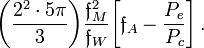$~\biggl(\frac{2^2\cdot 5 \pi}{3} \biggr) \frac{\mathfrak{f}_M^2}{\mathfrak{f}_W} \biggl[ \mathfrak{f}_A - \frac{P_e}{P_c} \biggr] \, .$

We have introduced these structural form factors into our discussion of the free energy of spherically symmetric, self-gravitating configurations because

Solution Strategies:

Detailed Force-Balance
(Introduction)

Virial Equilibrium
(Introduction)

 Example Solutions: Uniform-density sphere Isolated … ✓ ✓ Embedded in an External Medium … ✓ ✓ Polytropes Isolated … ✓ ✓ Embedded in an External Medium … ✓ ✓ Isothermal sphere Isolated … ✓ ✓ Embedded in an External Medium (Bonnor-Ebert Sphere) … ✓ ✓ Zero-temperature White Dwarf — Overview Power-law density distribution — Overview BiPolytropes (also referred to as Composite Polytropes) Overview Overview Core-Envelope Structure with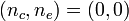$~(n_c,n_e) = (0,0)$ … ✓ ✓ Core-Envelope Structure with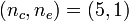$~(n_c,n_e) = (5,1)$ … ✓ ✓ Limiting Masses — Summary

### Stability:

 Solution Strategy Assuming Spherical Symmetry: Example Solutions:

### Dynamics:

 Spherical Collapse: Free Fall Homologous Collapse Collapse of Stellar Cores with n = 3 — investigation by Goldreich & Weber (1980, ApJ, 238, 991) Generalization to Arbitrary Polytropic Index

## Two-Dimensional Configurations

• Introduction

### Structure:

 Solution Strategies Axisymmetric Configurations Example Solutions: Maclaurin Spheroids Rotationally Flattened, Isothermal Structures Polytropic Tori: Papaloizou-Pringle (massless) Tori Self-gravitating Tori Infinitesimally Thin, Nonaxisymmetric Disk

## Three-Dimensional Configurations

• Introduction

### Structure:

 Solution Strategies Example Solutions: Ellipsoidal Figures of Equilibrium Compressible Analogs of Riemann Ellipsoids; see also Note to Hirschmann & Neilsen and Suggested Strategy by Joel